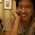## Saturday, April 14, 2012

### k-nearest neighbor search

A k-nearest neighbor search identifies the top k nearest neighbors to a query. The problem is: given a dataset D of vectors in a d-dimensional space and a query point x in the same space, find the closest point in D to x. The following function performs a k-nearest neighbor search using the euclidean distance:
```from numpy import random,argsort,sqrt
from pylab import plot,show

def knn_search(x, D, K):
""" find K nearest neighbours of data among D """
ndata = D.shape
K = K if K < ndata else ndata
# euclidean distances from the other points
sqd = sqrt(((D - x[:,:ndata])**2).sum(axis=0))
idx = argsort(sqd) # sorting
# return the indexes of K nearest neighbours
return idx[:K]
```
The function computes the euclidean distance between every point of D and x then returns the indexes of the points for which the distance is smaller.
Now, we will test this function on a random bidimensional dataset:
```# knn_search test
data = random.rand(2,200) # random dataset
x = random.rand(2,1) # query point

# performing the search
neig_idx = knn_search(x,data,10)

# plotting the data and the input point
plot(data[0,:],data[1,:],'ob',x[0,0],x[1,0],'or')
# highlighting the neighbours
plot(data[0,neig_idx],data[1,neig_idx],'o',
markerfacecolor='None',markersize=15,markeredgewidth=1)
show()
```
The result is as follows:

The red point is the query vector and the blue ones represent the data. The blue points surrounded by a black circle are the nearest neighbors.

1.How does this compare with using Scipy's cKDtree? http://docs.scipy.org/doc/scipy/reference/generated/scipy.spatial.cKDTree.html

2.Hi Michael, the class scipy.spatial.cKDTree implements another algorithm for the nearest-neighbor search based on KDTrees. Of course, KDTrees have pro and cons. For example, the search cost using a KDTree is logarithmic (so, it's faster than the naive algorithm implemented here) but you have to build the tree and if need to delete or insert points in your dataset, you have to modify the tree.
If you need more details look at this: http://en.wikipedia.org/wiki/K-d_tree

3.This comment has been removed by the author.

4."""Thanks a lot, I made a small change so that the user can make several queries with one call of knn_serach(...). Sorry I don't know how to format code here"""

from numpy import random,argsort,sqrt,array,ones
from pylab import plot,show

# The function computes the euclidean distance between every point of D and x then returns the indexes of the points for which the distance is smaller.
def knn_search(x, D, K):
""" find K nearest neighbours of data among D """
ndata = D.shape
# num of query points
queries=x.shape
K = K if K < ndata else ndata
# euclidean distances from the other points
diff=array(D*ones(queries,int)).T - x[:,:ndata].T
sqd=sqrt(((diff.T)**2).sum(axis=2))
# sorting
idx=argsort(sqd)
# return the indexes of K nearest neighbours
return idx[:,:K]

# Now, we will test this function on a random bidimensional dataset:
data = random.rand(200,2) # random dataset
x = array([[[0.4,0.4]],[[0.6,0.8]],[[0.9,0.2]],[[0.2,0.9]]]) # query points

# Performing the search
neig_idx = knn_search(x,data,10)

# Plotting the data and the input points
plot(data[:,0],data[:,1],'ob',x.T[0,0],x.T[1,0],'or')

# Highlighting the neighbours for each input
plot(data[neig_idx,0],data[neig_idx,1],'o', markerfacecolor='None',markersize=15,markeredgewidth=1)
#plot(data[neig_idx,0],data[neig_idx,1],'xk', markerfacecolor='None',markersize=15,markeredgewidth=1)
show()

5.Awesome code - this really helped me out! Thanks for sharing!

6.GP,

Great tutorial. Thanks as always for uploading these. One question, tho: It's not clear to me (a beginner) what form "x" should take when it's passed into knn_search function. You say that x is "a query point," but what does a query point look like?? Is it a slice of ndata -- a point within the features?? Thank you for your thoughts!

1.Hello, if you data matrix is of dimension n by m then x have to be a vector of dimension n.

2.Is data matrix, then, some kind of similarity or distance measure if I'm doing kNN on documents??

3.Usually each row of the data matrix contains one of your samples and the knn computes the distance between each sample you have and a query vector. At the end it reports to you the k samples closest to your query vector.

7.I have my training data in a csv file. The data contains 35 points corresponding to 3D vector in 3 columns x,y, and z and a feature 'color' in the fourth column. Not being a great pythonista, how do I modify your code here to employ my data to test a random new vector?

1.forgot to mention, the color feature is numeric 1, 0.5, 0.3, or 0. I want a new random vector to be predicted.

2.Hi, I would suggest you to read the CSV file using Pandas. Since your dataset has 3 dimensions you have to make a 3D plot (or ignore one of the variables). Matplotlib has a module named mplot3 that enables 3d visualization.

8.should be axis=1 instead of axis=0 for euclidean distance

1.9.Great post!

the only problem is, it only works for 2D spaces, while it would be much more usefull if it worked in higher dimentions!

1.the function knn_search should work also in higher dimension. If it doesn't work there's a bug.